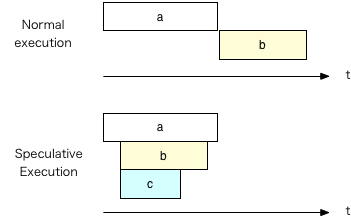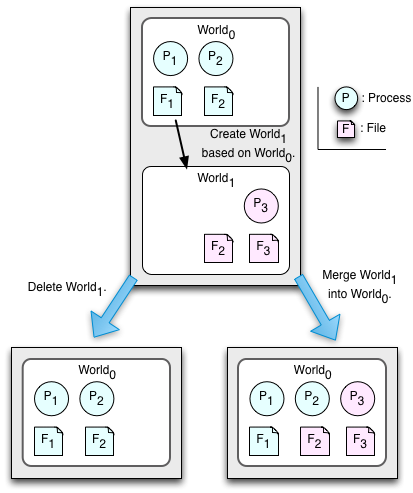# \$B%H%i%s%6%/%7%g%s!"(BArgus\$B!"Ej5!E*!&3Z4QE*

```\$BJB9T%7%9%F%`(B

\$B%7%9%F%`>pJs7O(B/\$B>pJs9)3X0h(B,
\$B%7%9%F%`>pJs9)3X8&5f72(B/\$B>pJsM}9)3X0L%W%m%0%i%`(B
\$B%7%9%F%`>pJs9)3X8&5f2J(B/\$B%3%s%T%e!<%?%5%\$%(%s%9@l96(B
\$B?7>k(B \$BLw(B
<yas@cs.tsukuba.ac.jp>
```

\$B\$3\$N%Z!<%8\$O!" ``` http://www.cs.tsukuba.ac.jp/~yas/cs/csys-2020/2020-07-03 ```
\$B\$"\$k\$\$\$O!" ` http://www.cs.tsukuba.ac.jp/~yas/cs/`
` http://www.cs.tsukuba.ac.jp/~yas/`

## \$B"#O"Mm;v9`(B

• \$B4|Kv;n83\$O9T\$\$\$^\$;\$s!#4|Kv%l%]!<%H\$b\$"\$j\$^\$;\$s!#Kh2s\$N%l%]!<%H\$G@.(B \$B@S\$rIU\$1\$^\$9!#(B There is no final exam and no final report. Grade are determined by individual class reports.
• \$B%l%]!<%H=P\$7\$F\$\$\$J\$\$?M\$O(B Web \$B%Z!<%8\$+\$i=P\$7\$F\$/\$@\$5\$\$!#(B You must submit reports via the Web page.
• \$B%l%]!<%H\$O!"(B7\$B7n(B14\$BF|(B \$B\$^\$G\$KA4ItDs=P\$7\$F\$/\$@\$5\$\$!#(B \$BDy@Z2a\$.\$?\$b\$N\$b!"Ds=P\$G\$-\$^\$9!#(B You must submit all the reports by July 14. You can submit reports after the deadlines.
• \$B0lIt!":FDs=P\$K\$J\$C\$F\$\$\$k\$b\$N\$,\$"\$j\$^\$9!#(B You may have some reports to post again.
• Twins\$B\$K\$"\$k2A%"%s%1!<%H(B \$B\$K2sEz\$*4j\$\$\$7\$^\$9!#(B \$B2sEz4|4V\$O!"(B7\$B7n(B2\$BF|\$+\$i(B7\$B7n(B14\$BF|\$G\$9!#(B Please answer the course evaluation questionnaire in Twins between July 2 and July 14.

## \$B"#:#F|\$N=EMW\$JOC(B

\$B#2\$D\$NAj(B
\$BBh#1Aj(B
\$BA4%W%m%;%9\$r(B Commit \$B\$b(B Abort \$B\$b\$G\$-\$k>uBV\$K\$9\$k!#(B
1. \$B%3!<%G%#%M!<%?\$O!"%m%0\$K!V=`Hw!W\$H=q\$/!#(B
2. \$B%3!<%G%#%M!<%?\$O!"%5%V%*!<%G%#%M!<%H\$K!V=`Hw!W%a%C%;!<%8\$rAw\$k!#(B
3. \$B%5%V%*!<%G%#%M!<%H\$O!"%m%0\$K!V=`Hw40N;!W\$H=q\$/!#(B
4. \$B%5%V%*!<%G%#%M!<%H\$O!"%3!<%G%#%M!<%?\$K!V=`Hw40N;!W%a%C%;!<%8\$rAw\$k!#(B
5. \$B%3!<%G%#%M!<%?\$O!"A4\$F\$N%5%V%*!<%G%#%M!<%H\$+\$i\$N1~Ez\$rBT\$D!#(B
\$BBh#2Aj(B
\$B
• \$B%3!<%G%#%M!<%?\$O!"%m%0\$K!V%3%_%C%H!W\$H=q\$/!#(B
• \$B%3!<%G%#%M!<%?\$O!"%5%V%*!<%G%#%M!<%H\$K!V%3%_%C%H!W%a%C%;!<%8\$rAw\$k!#(B
• \$B%5%V%*!<%G%#%M!<%H\$O!"%m%0\$K!V%3%_%C%H40N;!W\$H=q\$/!#(B
• \$B%5%V%*!<%G%#%M!<%H\$O!"%3!<%G%#%M!<%?\$K!V%3%_%C%H40N;!W%a%C%;!<%8\$rAw\$k!#(B
• \$B%3!<%G%#%M!<%?\$O!"A4\$F\$N%5%V%*!<%G%#%M!<%H\$+\$i\$N1~Ez\$rBT\$D!#(B
• \$B%/%i%C%7%e\$7\$?;~\$K\$O!"%m%0\$+\$i2sI|\$5\$;\$k!#(B

### \$B"!#2AX%3%_%C%H\$N@)Ls>r7o(B

`https://www.slideshare.net/kumagi/ss-78765920,\$BJ,;6%7%9%F%`\$K\$D\$\$\$F8l\$i\$;\$F\$/\$l(B by \$B7':j9(```` p32, 2 Phase Commit\$B\$O%P%0\$C\$F\$\$\$k(B \$B"!%H%i%s%6%/%7%g%sJ,N%%l%Y%k\$NNc(B ANSI/ISO SQL \$BI8=`\$GDj5A!#(BSerializable \$B\$O!"=E\$\$\$N\$G!"B?\$/\$N(B DBMS \$B\$G\$O%G(B \$B%U%)%k%H\$G\$OL58z!#(B <!-- TABLE.center-table TD { text-align:center; } TABLE.center-table TH { text-align:left; } --> \$BJ,N%%l%Y%k(B \$B%@!<%F%#!<%j!<%I(B \$B%N%s%j%T!<%?%V%k!&%j!<%I(B \$B%U%!%s%H%`!&%j!<%I(B Read Uncommitted \$BM-(B \$BM-(B \$BM-(B Read Committed \$BL5(B \$BM-(B \$BM-(B Repeatable Read \$BL5(B \$BL5(B \$BM-(B Serializable \$BL5(B \$BL5(B \$BL5(B \$B%@!<%F%#! \$B%N%s%j%T!<%?%V%k!&%j!<%I!#(B \$BB>\$N%H%i%s%6%/%7%g%s\$,@h\$K=*N;\$9\$k\$H!"ESCf\$GCM\$,JQ\$o\$C\$?\$h\$&\$K8+\$(\$k!#(B \$B%U%!%s%H%`!&%j!<%I!#4X78%G!<%?%Y!<%9\$G!"B>\$N%H%i%s%6%/%7%g%s\$G9T\$,(B \$BDI2C\$5\$l!"%3%_%C%H\$5\$l\$?;~!"(Bselect \$B\$N(B range \$B\$,JQ\$o\$C\$F\$7\$^\$C\$F8+\$(\$F\$7(B \$B\$^\$&!#(B \$B"#(BArgus \$B%H%i%s%6%/%7%g%s5!G=(B\$B\$rFbIt\$K4^\$`8@8l!#(B \$BH/2;\$O!"1Q8lFI\$_\$G%"!<%,%9!#%.%j%7%c?@OCFI\$_\$G\$O!"%"%k%4%9!#(B Barbara Liskov: "Distributed programming in Argus", Communications of the ACM, Vol.31, No.3, pp.300-312, 1988. https://doi.org/10.1145/42392.42399 \$BJ8K!\$O!"(BCLU \$B8@8l\$K;w\$F\$\$\$k!#(B \$B;29M(B: http://www2.gssm.otsuka.tsukuba.ac.jp/staff/kuno/lectures/,(\$B%j%s%/@Z\$l(B) 1993.5 \$B%<%_(B: CLU\$B8@8lF~Lg(B@GSSM ( http://160.16.241.20/lectures/,\$B5WLn\$N9V5A!&9V1i!&%W%l%<%s;qNA(B/\$B9V5A;qNA(B ) http://www.pmg.lcs.mit.edu/CLU.html CLU Home Page. \$B"!%,!<%G%#%"%s(B(guardian) \$BJB9T\$KF0:n\$9\$k%*%V%8%'%/%H(B(\$B8=:_\$NMQ8l\$G\$O%9%l%C%I(B)\$B\$r \$B%O%s%I%i(B(handler)\$B\$H8F\$P\$l\$k(B \$BB>\$N%,!<%G%#%"%s\$+\$i8F\$V\$3\$H\$,\$G\$-\$k \$B=i4|2=MQ\$K%/%j%(!<%?(B(creator)\$B\$H8F\$P\$l\$k%(%s%H%j\$r;}\$D!#(B \$B"!0BDj\$JJQ?t(B(Stable variable) \$B%,!<%G%#%"%s\$O!"#2 \$B0BDj(B(stable) \$B%/%i%C%7%e!J:F5/F0!K\$7\$F\$b!"@8\$-;D\$k!#(B \$B4xH/(B(volatile) \$B%/%i%C%7%e!J:F5/F0!K\$9\$k\$H!"CM\$,<:\$o\$l\$k!#(B \$B"!%"%/%7%g%s(B(action) \$B0BDj\$JJQ?t\$KBP\$9\$k%H%i%s%6%/%7%g%s\$ruBV\$+\$i!"uBV\$XA+0\\$5\$;\$k!#(B( \$BESCf\$G%/%i%C%7%e\$7\$?\$i!"85\$KLa\$9!#(B) \$B%H%C%W%"%/%7%g%s(B \$B%H%C%W%l%Y%k\$N%H%i%s%6%/%7%g%s\$r \$B%5%V%"%/%7%g%s(B \$B%M%9%H\$7\$?%H%i%s%6%/%7%g%s\$r \$BB>\$N%,!<%G%#%"%s\$N%O%s%I%i\$N8F=P\$7(B(\$B1s3V\$B"!(BArgus\$B\$K\$*\$1\$k%H%i%s%6%/%7%g%s\$N \$B#2Aj%3%_%C%H(B \$B=q9~\$_%m%C%/\$G!":n6HMQ\$N%3%T!<\$,:n\$i\$l\$k!#(B \$B"!%,!<%G%#%"%s\$N@k8@(B/Declaration of guardians \$BL>A0(B Name \$B@8@.%k!<%A%s!#0z?t\$N \$B0BDj\$JJQ?t!#(BStable variables \$B4xH/E*\$JJQ?t!#(BVolatile variables \$B%O%s%I%i \$B%P%C%/%0%i%s%I!&%k!<%A%s!#%,!<%G%#%"%s<+?H\$N%W%m%0%i%`!#(B Backgroud routines. \$BI|5luBV\$KLa\$9!#(B Recovery procedures. \$B0BDj\$JJQ?t\$K\$D\$\$\$F\$O!"%7%9%F%`\$,<+F0E*\$K@09g@-\$,uBV\$KI|5l\$9\$k!#(B \$B"!6d9T\$N;YE9(B/A bank branch in Argus 1: branch = guardian is create handles total, open, close, deposit, withdraw 2: % type definitions 3: htable = atomic_array[bucket] 4: bucket = atomic_array[pair] 5: pair = atomic_record[num: account_number, acct: acct_info] 6: acct_info = atomic_record[bal: int] 7: account_number = atomic_record[code: string, num: int] 8: intcell = atomic_record[val: int] 9: 10: stable ht: htable % the table of accounts 11: stable code: string % the code for the branch 12: stable seed: intcell % the seed for generating new account numbers 13: 14: create = creator (c: string, size: int) returns (branch) 15: code := c 16: seed.val := 0 17: ht := htable\$new() 18: for i: int in int\$from_to(1, size) do 19: htable\$addh(ht, bucket\$new()) 20: end 21: return (self) 22: end create 23: 24: total = handler () returns (int) 25: sum: int := 0 26: for b: bucket in htable\$elements(ht) do 27: for p: pair in bucket\$elements(b) do 28: sum := sum + p.acct.bal 29: end 30: end 31: return (sum) 32: end total 33: 34: open = handler () returns (account_number) 35: intcell\$write_lock(seed) % get a write lock on the seed 36: a: account_number := account_number\${code: code, num: seed.val} 37: seed.val := seed.val + 1 38: bucket\$addh(ht[hash(a.num)], pair\${num: a, acct: acct_info\${bal: 0}}) 39: return (a) 40: end open 41: 42: close = handler (a: account_number) signals (no_such_acct, positive_balance) 43: b: bucket := ht[hash(a.num)] 44: for i: int in bucket\$indexes(b) do 45: if b[i].num != a then continue end 46: if b[i].acct.bal > 0 then signal positive-balance end 47: b[i] := bucket\$top(b) % store topmost element in place of closed account 48: bucket\$remh(b) % discard topmost element 49: return 50: end 51: signal no_such_acct 52: end close 53: 54: lookup = proc (a: account_number) returns (acct_info) signals (no_such__acct) 55: for p: pair in bucket\$elements(ht[hash(a.num)]) do 56: if p.num = a then return (p.acct) end 57: end 58: signal no_such_acct 59: end lookup 60: 61: deposit = handler (a: account_number, amt: int) signals (no_such_acct, 62: negativ_amount) 63: if amt < 0 then signal negative_amount end 64: ainfo: acct_info := lookup(a) resignal no_such_acct 65: ainfo.bal := ainfo.bal + amt 66: end deposit 67: 68: withdraw = handler (a: account_number, amt: int) signals (no_such_acct, 69: negative_amount, insufficient_funds) 70: if amt < 0 then signal negative amount end 71: ainfo: acct_info := lookup(a) resignal no_such_acct 72: if ainfo.bal < amt then signal insufficient_funds end 73: ainfo.bal := ainfo.bal - amt 74: end withdraw 75: end branch \$B%,!<%G%#%"%s\$rDj5A\$7!"(Bbranch \$B\$H\$\$\$&JQ?t\$K%P%\$%s%I(B \$B%/%j%(!<%?\$O!"(Bcreate() \$B%O%s%I%i\$O!"(Btotal(), open(), close(), deposit(), withdraw() \$B7?Dj5A!#(Brecord \$B\$O!"9=B\$BN\$NDj5A!#(B atomic \$B\$J\$N\$G!"%7%9%F%`\$K\$h\$j%H%i%s%6%/%7%g%s\$NBP>]\$H\$J\$k!#(B \$BJQ?tDj5A!#(Bstable \$B\$J\$N\$G!"A4It0BDj!#(B \$B4xH/E*\$JJQ?t\$,\$J\$\$\$N\$G!"%/%i%C%7%e\$+\$i\$N2sI|%3!<%I\$O\$J\$\$!#(B \$B%P%C%/%0%i%s%I\$N seed \$B\$O!"8}:BHV9f\$r%f%K!<%/\$K\$9\$k\$?\$a\$N%+%&%s%?!#(B \$B"#(BEtherium-Solidity Etherium \$B\$O!"%V%m%C%/%A%'!<%s5;=Q\$N0l \$B%"%+%&%s%H\$O!"(Baddress \$B\$r;}\$D!#(B \$B%"%+%&%s%H\$O!"uBV\$r;}\$D!#(B balance (\$B;D9b(B) \$B%"%+%&%s%H\$K!"(B2\$B EOA\$B!J(BExternally Owned Account)\$B!#8D?M\$N:bI[!#?M\$KI3IU\$\$\$F\$\$\$k!#(B \$B8x3+80!&HkL)80\$NAH\$r;}\$D!#(B Contract Account\$B!#(BSmart contract\$B!#%V%m%C%/%A%'!<%s\$KIU?o\$7\$?2>A[7W(B \$B;;5!\$GuBV\$r;}\$D!#%V%m%C%/%A%'!<(B \$B%s!&%M%C%H%o!<%/\$K%G%#%W%m%\$\$5\$l\$k!#(B EOA \$B\$+\$iB>\$N(B EOA\$B!"\$^\$?\$O!"(BEOA \$B\$+\$i(B Contract Account \$B\$K(B ether \$BCM\$rAw?.\$9\$k\$3\$H\$r(B Etherium \$B\$G\$O!"(B \$B%H%i%s%6%/%7%g%s\$H8@\$C\$F\$\$\$k!#(B \$B%H%i%s%6%/%7%g%s\$O!" to: \$B data: \$B%Z%\$%m!<%I(B value: \$BAw?.\$5\$l\$k(B ether \$BCM(B nonce: \$BAw?. gasprice, gasLimit, starttagas: \$B (v,r,s): \$B%G%#%8%?%k=pL>(B Contract Account\$B\$+\$iH/?.\$5\$l\$k%H%i%s%6%/%7%g%s\$O!"%a%C%;!<%8\$H8F\$P\$l\$k!#(B \$B%H%i%s%6%/%7%g%s\$G!"Fs=E;YJ'\$\$\$,5/\$-\$J\$\$\$h\$&\$K!"(Bether \$B\$,A}\$(\$?\$j8:\$C(B \$B\$?\$j\$7\$J\$\$\$h\$&\$K!"%V%m%C%/%A%'!<%s\$N%N!<%I(B(\$B%^%\$%J!<(B)\$B\$,4F;k\$7\$F\$\$\$k!#(B \$B%H%i%s%6%/%7%g%s\$N Contract \$B\$N4X?t\$N \$B%H%i%s%6%/%7%g%s\$G\$O!"(Bcontract \$B\$K!"(Bether \$B\$r%H%i%s%6%/%7%g%s\$GAw\$j!"(B Contract \$BFbIt\$N>uBV\$rJQ2=\$5\$;\$k\$3\$H\$,\$G\$-\$k!#>uBV\$NJQ2=\$O!"%V%m%C%/%A%'!<(B \$B%s\$K5-O?\$5\$l\$k!#(B \$B8F\$S=P\$7\$G\$O!"(Bcontract \$B\$N>uBV\$rJQ2=\$5\$;\$i\$l\$J\$\$!#%H%i%s%6%/%7%g%s(B \$B\$G\$O\$J\$\$\$N\$G!"(B(\$B7Z\$\$\$b\$N\$O(B)\$BuBV\$r=8\$a\$F!"%3!<%I\$r!"%m!<%+%k\$G \$B"!6d9T\$N8}:B(B/A bank account in Solidity contract Bank { mapping(address => uint256) public accounts; function deposit() { accounts[msg.sender] += msg.value; } function withdraw(uint256 amount) { if(amount > accounts[msg.sender]) throw; accounts[msg.sender] -= amount; if(!msg.sender.send(amount)) throw; } function get_balance() { return accounts[msg.sender]; } } mapping \$B\$O!"%O%C%7%eI=\$N\$h\$&\$J\$b\$N!#\$3\$NNc\$G\$O!"%-!<\$,(B address \$B\$G!"(B \$BCM\$,(B uint256\$B!#JQ?tL>\$,(B accounts\$B!#(Baddress \$B\$O!"%"%+%&%s%H\$N%"%I%l%9!#(B msg \$B\$O!"%H%i%s%6%/%7%g%s\$GAw\$i\$F\$-\$?%a%C%;!<%8!#(B \$B msg.sender: \$BAw?. msg.value: \$BAw\$i\$l\$F\$-\$?(B ether \$B\$NCM!#C10L\$O(B wei (ether / 1e18)\$B!#(B deposit() \$B\$O!"Aw?.85\$N%"%+%&%s%H\$+\$i(B amount \$BJ,\$N(Bether \$B\$r \$B \$B\$3\$N%H%i%s%6%/%7%g%s\$G!"(Bcontract \$B\$,;}\$C\$F\$\$\$k(B balance \$B\$b!"A}\$(\$k!#(B withdraw() \$B\$O!"%a%C%;!<%8\$NAw?.85\$K!"MB\$+\$C\$F\$\$\$k(B ether \$B\$r(B amount \$BJ,\$@\$1JV\$9!#(B require() \$B\$,@.\$jN)\$?\$J\$\$\$H!"%H%i%s%6%/%7%g%s\$O!"(Babort \$B\$5\$l\$k!#(B \$B\$^\$:!"(Baccounts[msg.sender]\$B!!\$r8:\$i\$9!#@h\$K8:\$i\$9\$N\$,Bg;v!#(B \$B \$B\$3\$N%H%i%s%6%/%7%g%s\$G!"(Bcontract \$B\$,;}\$C\$F\$\$\$k(B balance \$B\$,8:\$k!#(B \$B;D9b\$r3NG'\$7!"@h\$K(B accounts[msg.sender] \$B\$r8:\$i\$7\$F\$+\$i%H%i%s%6%/%7%g%s(B \$B\$r9T\$o\$J\$\$\$H!"J#?tF1;~\$K(B withdraw() \$B\$,8F\$P\$l\$?;~\$K!"LdBj\$,@8\$8\$k!#(B get_balance() \$B\$O!";D9b>H2q!#(B \$B>uBV\$rJQ\$(\$J\$\$\$N\$G!"%H%i%s%6%/%7%g%s\$G\$O\$J\$/(B call \$B\$G\$B"#Ej5!E* \$BEj5!E*-MhMxMQ\$5\$l\$k\$+MxMQ\$5\$l\$J\$\$\$+3NDj\$5(B \$B\$l\$kA0\$K if( a() ) { b(); } else { c(); } \$BIaDL\$O!"(Ba() \$B\$,(B true \$B\$+(B false \$B\$+\$rJV\$9\$N\$rBT\$C\$F\$+\$i(B b() \$B!"\$^\$?\$O!"(Bc() \$B\$r3+;O\$9\$k!#(B \$BEj5!E*=hM}\$G\$O!"(Ba() \$B\$N40N;\$rBT\$?\$:\$K!"@h\$K!"(Bb()\$B!"\$^\$?\$O!"(Bc() \$B\$^\$?(B \$B\$O!"(Bb() \$B\$H(B c() \$B\$NN>J}\$r\$B?^(B? \$BEj5!E* \$B\$3\$NNc\$G\$O!"(Ba() \$B\$,40N;\$7\$?;~\$K\$O!"(Bb()\$B!"(Bc() \$B\$,=*\$o\$C\$F\$\$\$?;~\$K\$O!"8+\$+\$1(B \$B>e!"B(:B\$K=*\$o\$k!#(B \$B8=:_\$N(BCPU\$B\$O!"5!3#8lL?Na\$NC10L\$GEj5!E* \$B:G6a\$N(BCPU\$B\$O!"J,4tM=B,\$r\$7\$F!">r7oJ,4tL?Na\$N@h\$^\$G@h\$Kr(B \$B7o\$,K~\$?\$5\$l\$J\$\$;~\$K\$O7k2L\$O \$B4X?tC10L!"JEENO\$H\$OAjH?\$9\$k!#(B \$B4X?tC10L!" then \$BIt\$d(B else \$BIt\$N=hM}\$K!"I{:nMQ\$,\$J\$\$(B(\$B4X?t7?(B)\$B\$N;~\$K\$O!"(B \$B \$BI{:nMQ\$,\$"\$k;~\$K\$O!"%H%i%s%6%/%7%g%sE*\$J \$B"!@\$3&(BOS/World OS \$B?7>k\$N8&5f@.2L!#(B \$B:,O)LC(B, \$BaDbC(B, \$B?7>k(B, \$B4n20Ip(B, \$B2'D9(B: "\$BAFN3EYEj5!E*JBNs=hM}\$r;Y1g(B \$B\$9\$k%*%Z%l!<%F%#%s%0!&%7%9%F%`\$N9=A[(B", SWoPP'94, \$B>pJs=hM}3X2q8&5f2qJs(B \$B9p(B94-ARC-107-12, Vol.94,No.66, pp.89-96 (1994\$BG/(B7\$B7n(B). Jun Sun, Yasushi Shinjo and Kozo Itano: "The Implementation of a Distributed File System Supporting the Parallel World Model", The Third International Workshop on Advanced Parallel Processing Technologies, pp.43-47 (Oct.19-21, 1999). \$B@P0f(B \$B9'1R(B, \$B?7>k(B \$BLw(B, \$BHDLn(B \$B9N;0(B: "\$B%W%m%;%9%H%l!<%95!G=\$rMQ\$\$\$?@\$3&(B OS\$B\$NpJs=hM}3X2qO@J8;o(B, Vol.43, No.6, pp.1702-1714 (2002\$BG/(B6\$B7n(B). \$B:#N\$K.IW(B, \$BNkLZ??0l(B, \$B?7>kLw(B, \$BHDLn9N;0(B: "\$BF0E*%j%s%/\$H%Q%1%C%H%U%#%k(B \$B%?\$rMQ\$\$\$?@\$3&(BOS \$B\$N%M%C%H%o!<%/5!G=\$N Yasushi Shinjo, Wataru Ishida and Jinpeng Wei: "Implementing a parallel world model using Linux containers for efficient system administration", IEEE Second International Workshop on Container Technologies and Container Clouds (WoC 2016), 7 pages (2016). SF (Science Fiction) \$B\$N%Q%i%l%k%o!<%k%I(B(parallel world, parallel universe)\$B\$r!"%*%Z%l!<%F%#%s%0!&%7%9%F%`\$N%l%Y%k\$G\$B?^(B SF\$B\$N%Q%i%l%k%o!<%k%I(B \$B#S#F\$G\$O!";~!9JL\$N@\$3&\$K%8%c%s%W\$7\$?\$j?M\$,F~\$lBX\$o\$C\$?\$j\$9\$k!#(B \$B"!(BUndo \$B\$G\$-\$k(BOS/Undoable OS Undo \$B\$H\$O!"(Bdo \$B\$N8z2L\$rBG\$A>C\$7\$F!"\$b\$H\$b\$H(B do \$B\$7\$J\$+\$C\$?\$3\$H\$K\$9\$k!#(B (do not \$B\$H\$O0c\$&!#(B) \$B@\$3&#O#S\$G\$O!"%U%!%\$%k\$NFbMF\$rJQ99\$9\$k\$H!"#2\$D\$N@\$3&\$,\$G\$-\$k!#(B \$BJQ99\$5\$l\$?@\$3&(B \$BJQ99\$5\$l\$F\$\$\$J\$\$@\$3&(B\$B?^(B \$B@\$3&(BOS\$B\$G;H\$(\$k@\$3&\$NA`:n(B \$B \$BIaDL\$N2>A[7W;;5!\$G\$O!"M;9g\$O\$G\$-\$J\$\$!#7Q>5\$N%3%9%H\$,Bg\$-\$\$!#(B \$B"!%Q%i%l%k%o!<%k%I\$NMxMQ(B/Usage of parallel worlds \$B%/%i%C%/%-%s%0!"967b\$K\$h\$kGK2u\$+\$i\$N?WB.\$J2sI|!#967b\$5\$l\$k(B \$B2DG=@-\$,\$"\$k%W%m%0%i%`!"(B \$B2x\$7\$\$%W%m%0%i%`\$r;R@\$3&\$G \$B>.5,LO\$J%5!<%P\$N4IM}!#%Q%C%AEv\$F\$r!";R@\$3&\$G \$BEj5!E*=hM}!?3Z4QE*=hM}!# \$B!V:9J,!W\$r(B first class object \$B\$H\$7\$F07\$&!#(B \$BJ#?t\$N#P#C\$G\$N%U%!%\$%kFbMF\$NE}0l!#J#?t\$N@\$3&\$NFbMF\$rHf3S\$7\$F(B \$B99?7\$5\$l\$?ItJ,\$rH?1G\$5\$;\$k!#(B A difference as a first class object. \$B"!(BHOPE C. Cowan and H. L. Lutfiyya: "A wait-free algorithm for optimistic programming: Hope realized", In Proceedings of the 16th International Conference on Distributed Computing Systems(ICDCS), pages 484-493, 1996. RPC\$B\$NCY1d\$r1#\$9!#(B This paper proposes hiding RPC delays by using optimistic execution. \$B"#2A%"%s%1!<%H(B/Course Evaluation Questionnaire CS\$B@l96!&>pJsM}9)3X0L%W%m%0%i%`\$N653X%^%M%8%a%s%H0Q0w2q\$G\$O!"(B \$B650i\$N2~A1\$N\$?\$a\$K!"3X@8\$N3'\$5\$s\$K2A%"%s%1!<%H\$r \$B%"%s%1!<%H\$O(BTWINS\$B\$+\$i2sEz\$7\$F\$/\$@\$5\$\$!#(B \$B\$J\$*!"3'\$5\$s\$NI>2A\$,@.@S\$K1F6A\$9\$k\$3\$H\$O0l@Z\$"\$j\$^\$;\$s!#(B \$B\$^\$?!"I>2A7k2L\$r650i\$N2~A10J30\$NL\E*\$KMxMQ\$9\$k\$3\$H\$O\$"\$j\$^\$;\$s\$7!"(B \$BI>2A7k2L\$r8x3+\$9\$k>l9g\$K\$O8D?M\$rFCDj\$G\$-\$k\$h\$&\$J>pJs\$O4^\$a\$^\$;\$s!#(B \$B%"%s%1!<%H\$O!" \$BJB9T%7%9%F%`(B \$BJB9T%7%9%F%`(B-\$B8D!9\$N2s\$4\$H\$NI>2A(B \$B2sEz4|4V\$O!"(B7\$B7n(B2\$BF|\$+\$i(B7\$B7n(B14\$BF|\$G\$9!#(B The FD Committee of the Department of Computer Science/Master's and Doctoral Programs in Computer Science conducts a survey of all students for the purpose of evaluating and improving instruction. Please complete this questionnaire in TWINS. These answers will not affect your academic grades. The result will not be used for any purpose other than instruction evaluation and its further improvement. When the summarized information of this questionnaire is released to the public (for FD activities), no private information about you will be included. \$B"#N}=,LdBj(B(exercise)10 \$B%H%i%s%6%/%7%g%s!"(BArgus\$B!"Ej5!E*!&3Z4QE* \$B!zLdBj(B(1001) \$B%m%C%/\$H\$NHf3S(B/Comparisons with locking \$B%m%C%/\$r;H\$&J}K!\$HHf3S\$7\$F!"%H%i%s%6%/%7%g%s\$rMQ\$\$\$kJ}K!\$N=EMW\$JMxE@\$r(B \$B<(\$7\$J\$5\$\$!#(B Write an important advantage of using transaction over using locking. \$B!zLdBj(B(1002) Argus Argus \$B8@8l\$N%,!<%G%#%"%s\$H Concurrent Pascal \$B\$N%b%K%?(B Monitors in Concurrent Pascal Java \$B\$N(B synchronized \$B\$K\$h\$k%b%K%?(B Monitors by "synchronized" in Java Pthread \$B\$N(B mutex \$B\$K\$h\$k%b%K%?(B Monitors by mutex in C Pthread \$B!zLdBj(B(1003) \$BEj5!E* \$BEj5!E* Last updated: 2020/07/01 21:25:47 Yasushi Shinjo / <yas@cs.tsukuba.ac.jp> ```